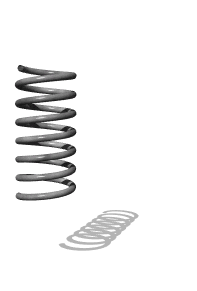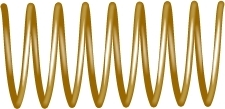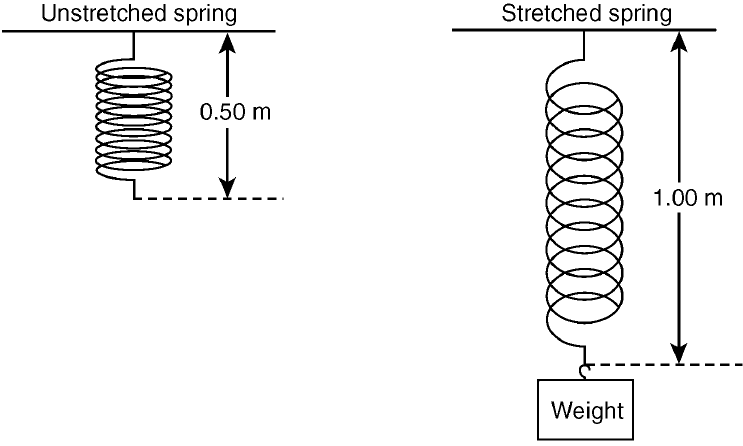﻿ Elastic PE and K NotesHomeTry this at home!

Egg trick learn how now

showmethephysics.com

PE and k

e) PEs in terms of k - Ideal Spring

1. P.E.s = Fx

Average Force:  F = F/2

2.  PE = (F/2)x

substitute  F = Kx (On reference)

3.  P.E.s = (1/2)(Kx)x

 P.E.s = 1/2(kx2)

(On reference)

 Ex 1) A force of 12. N stretches a spring and makes it .15m longer a) What the spring constant (k) of this spring?Ex 1) A force of 12. N stretches a spring and makes it .15m longer

a) What's the spring constant (k) of this spring?

k = F/x

= 12. N/.15 m

= 80. N/mb) P.E. of this spring?

PEs = (½)Kx2

= 1/2(80. N/m)(.15 m2)

Be sure to use parenthesis when calculating!!

PEs = .90 JoulesEx 2) If 15. joules of energy are stored in the stretched spring below, what is the value of the spring constantEx 2) If 15. joules of energy are stored in the stretched spring, what is the value of the spring constantP.E.s = 1/2(kx2)

15. J = (½)k(.50 m2)

1/2 in decimal form is .50

15. J = .50 k(.25)

15  = .125 k

Divide both sides by .125

k = 120 N/m

 What's wrong with this picture?   P.E.s = (1/2)kx2 x = elongationP.E.s = 1/2(kx2) PE, X direct square Plot should be curvedKinetic Energy